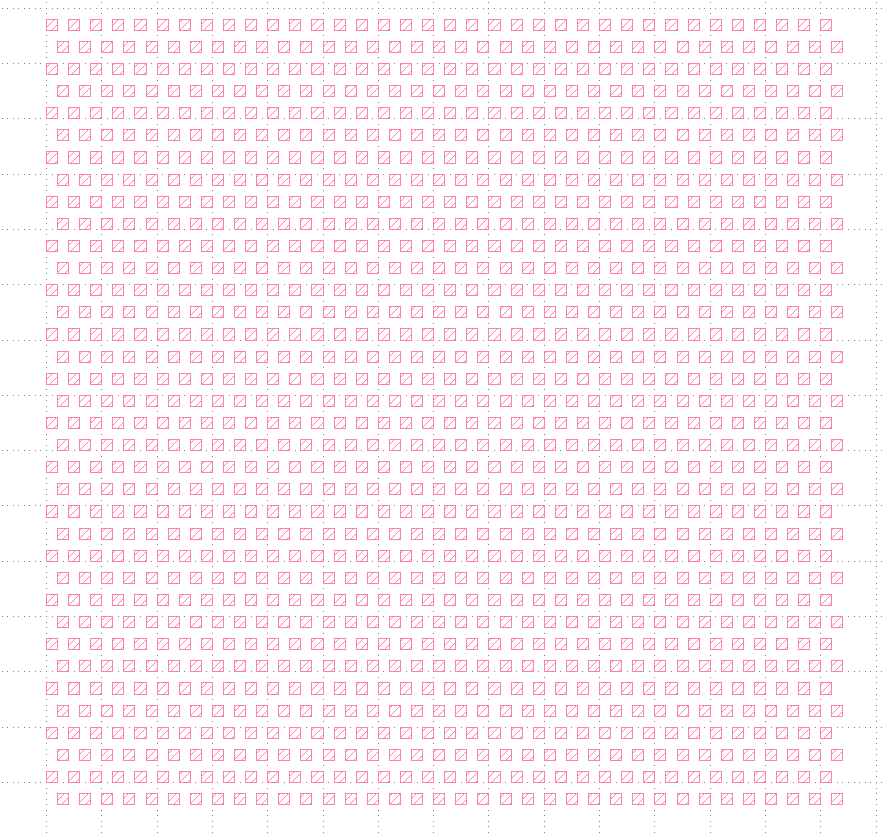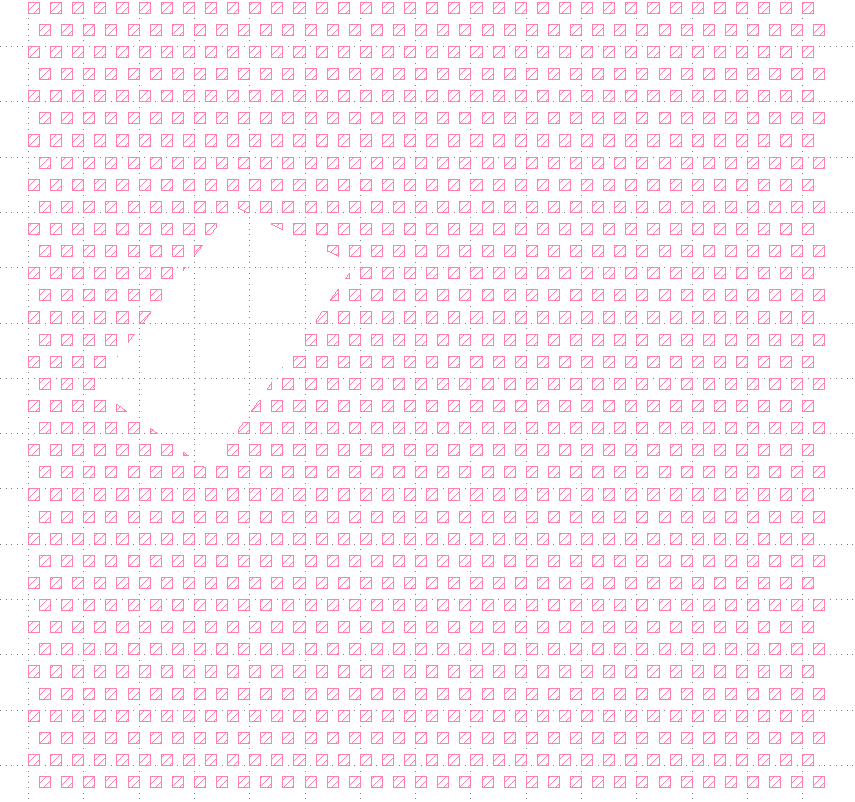# Some question about 'erase' in python

Hi

I am using Python scrfipting in marco develpoment to draw patterns. I need to make a ''customized eraser". So I tried the 'erase' function. But it seems like it can only delete an existing obiect that is inserted.

And when I tried a cuntomized shape like:
erase(pya.Box(x1, y1, x1+length, y1+width1)) it gives that: 'erase' is not defined

when I tried:
cell.shapes(layer).erase(pya.Box(x1, y1, x1+length, y1+width1)) it gives: unexpected object type.

It only works when I tried:
cell.shapes(layer).erase(cell.shapes(layer).insert(pya.Box(x1, y1, x1+length, y1+width1)))

But this does not help, because I need to clear everything which is surrounded by a customized shape.

So how to make a general customized eraser or is there any other function to realize my purpose?

• It is beacuse my pattern has a background, I need to clear some certain parts in order to fit my structure in it. And it dose not help to just erase the background by hand in Editor mode.

• Hi,

I think you misunderstood the meaning of "erase": it will take out a specific object from the object collection, not void the area under the shape.

What you need is a "boolean NOT" operation. Or you need a selective delete. "NOT" is available within the DRC framework, but it will not preserve the hierarchy not labels of the original layer. A selective delete is a difficult operation if hierarchy is involved and shapes need to be deleted from subcells.

I can't give advise without knowing more about the application you're planning.

Matthias

• edited March 11

Hi Matthias

This is my script. I have made the certain shape for the 'eraser' and the background. What I need to do is something like from image 1 to image 2.I think it is really difficult to use the 'boolean' operator for my case. It can be much easier if there is a function to customize the shape of 'erase' in Editor mode.

# script

``````def block(layout, cell, layer, width, x0, y0,rad,x_r, y_r, width1, length, x1, y1, r):

theta_start =0
theta_end =360
theta = np.linspace(theta_start, theta_end, 800)
a1=[]
for i in range(len(theta)):
a1.append(pya.Point(x[i], y[i]))
wg_path1=pya.Path(a1,width1)

theta_start=0  #angle in degree
theta_end = 90  #angle in degree
theta = np.linspace(theta_start, theta_end, 200)
x2 = r*np.cos(2*np.pi*theta/360)+(x1+length);
y2 = r*np.sin(2*np.pi*theta/360)+ (y1-r+width1/2);
a2 = []
for i in range(len(theta)):
a2.append(pya.Point(x2[i], y2[i]))
wg_path2 = pya.Path(a2, width1)    #turing waveguide
theta_start=180
theta_end = 270
theta = np.linspace(theta_start, theta_end, 200)
x = r*np.cos(2*np.pi*theta/360)+(x1+length+2*r);
y = r*np.sin(2*np.pi*theta/360)+ (y1-r+width1/2);
a3 = []
for i in range(len(theta)):
a3.append(pya.Point(x[i], y[i]))
wg_path3 = pya.Path(a3, width1) #turing waveguide

wg_path4=pya.Box(x1, y1, x1+length, y1+width1)  #straight waveguide

eraser=[wg_path1,wg_path2,wg_path3,wg_path4]    #the eraser is a combination of four parts

for o in range(2):
for u in range(18):
for i in range(36):
hull=pya.Box(x0+(2*i+o)*width, y0+(4*u-2*o)*width,x0+(2*i+1+o)*width, y0+(1+4*u-2*o)*width)
cell.shapes(layer).insert(pya.Polygon(hull))  # background
if  __name__ ==  "__main__" :

layout = pya.Layout()
top = layout.create_cell("TOP")
l1 = layout.layer(1, 0)
y0_1=0
x0_1=0
x1_1=1000
y1_1=1000
width_1=10000
width_2=10000
length_1=800000
r_1=40000
x_r1=length_1/2
block(layout=layout, cell=top, layer=l1, width=width_1,  x0=x0_1, y0=y0_1,rad=rad_1, x_r=x_r1, y_r=y_r1, width1=width_2, length=length_1, x1=x1_1, y1=y1_1, r=r_1)
layout.write("D:/cmp_block.gds")
print("Done.")
``````

Thank you

• Hi,

Please format your code using Markdown formatting - put a line with a triple backtick before and after the code. The above form is not quite readable.

I think boolean operations are basically the easiest way to implement your request. Your script is already producing a layout with the "erase" layer.

You can then use a DRC script like this:

``````# This will remove the "eraser" from layer 17, datatype 0 (example):
eraser = layout("D:/cmp_block.gds")

(input(17,0) - eraser.input(1, 0)).output(17, 0)
``````

If you care to write your script in Ruby you can basically integrate the eraser generation into the DRC script and skip the intermediate file step.

Matthias

• Hi Matthias,

I finally find the function 'Region'. Just use `pya.Region(A)-pya.Region(B)`, then it gives what I need. And this is what so called 'boolean'. Because I am a noob of coding, it really took me a while to understand how it goes.
Anyway, thank you for being so helpful.

Zhan

• Hi Zhan,

very good ... that is actually even better. I did not dare to propose this, but this will allow you to implement the feature in a single piece of code.

"Regions" are basically what is a "layer" on the canvas. The region object allows manipulating and analysing layers in manifold ways. It's a very mighty concept.

I just would like to point out this:

• Regions don't represent labels (text objects). Hence such objects will be lost.
• Regions only know polygons. Paths will be converted into polygons if you use regions. Same for boxes.
• Regions are flat. If you have a hierarchy of cells, the Regions will represent the flattened view (as looking from the top cell). Boolean operations will then give you the full layer, but the hierarchical composition is lost.

Regards,

Matthias